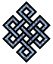#fail2ban bad ip database: ip 122.166.186.167

### | ip database | live view | stats | report | help | api key:

 ip: 122.166.186.167 hostname: abts-kk-static-167.186.166.122.airtelbroadband.in country:[IN] India first reported: 17.11.2018 12:14.27 GMT+0200 last reported: 01.12.2018 18:35.41 GMT+0200 time period: 14d 06h 21m 14s total reports: 11 reported by: 2 host(s) filter(s): ssh (6) ssh (5) tor exit node no badips.com db Lookup## port scan of '122.166.186.167':

[-hide]
```# Nmap 6.40 scan initiated Sat Nov 17 12:15:03 2018 as: /usr/bin/nmap -sU -sS -O 122.166.186.167
Nmap scan report for abts-kk-static-167.186.166.122.airtelbroadband.in (122.166.186.167)
Host is up (0.15s latency).
Not shown: 1000 open|filtered ports, 989 filtered ports
PORT     STATE  SERVICE
21/tcp   open   ftp
22/tcp   open   ssh
80/tcp   open   http
443/tcp  open   https
3306/tcp open   mysql
5001/tcp closed commplex-link
5002/tcp closed rfe
5003/tcp closed filemaker
5004/tcp open   avt-profile-1
8009/tcp open   ajp13
8080/tcp open   http-proxy
No exact OS matches for host (If you know what OS is running on it, see http://nmap.org/submit/ ).
TCP/IP fingerprint:
OS:SCAN(V=6.40%E=4%D=11/17%OT=21%CT=5001%CU=%PV=N%G=Y%TM=5BEFF860%P=x86_64-
OS:pc-linux-gnu)SEQ(SP=106%GCD=1%ISR=10A%TI=I%CI=I%TS=U)SEQ(SP=106%GCD=2%IS
OS:R=10A%TI=I%TS=U)OPS(O1=M5B4NW8NNS%O2=M5B4NW8NNS%O3=M5B4NW8%O4=M5B4NW8NNS
OS:%O5=M5B4NW8NNS%O6=M5B4NNS)WIN(W1=FFFF%W2=FFFF%W3=FFFF%W4=FFFF%W5=FFFF%W6
OS:=FF70)ECN(R=Y%DF=Y%TG=80%W=FFFF%O=M5B4NW8NNS%CC=N%Q=)T1(R=Y%DF=Y%TG=80%S
OS:=O%A=S+%F=AS%RD=0%Q=)T2(R=N)T3(R=N)T4(R=N)T5(R=Y%DF=Y%TG=40%W=0%S=Z%A=S+
OS:%F=AR%O=%RD=0%Q=)T6(R=Y%DF=Y%TG=40%W=0%S=A%A=Z%F=R%O=%RD=0%Q=)T7(R=N)U1(
OS:R=N)IE(R=N)

OS detection performed. Please report any incorrect results at http://nmap.org/submit/ .
# Nmap done at Sat Nov 17 12:15:44 2018 -- 1 IP address (1 host up) scanned in 42.94 seconds
```
```Σ = 42 | Δt = 0.0054299831390381s
```